Maths-
General
Easy

Question

# If 18 binders can bind 900 books in 10 days, how many binders will be required to bind 660 books in 12 days?Hint:

## The correct answer is: 12 days.

### Let the number of binders be represented as x, the number of books be represented as y and the number of days be represented as z. Now, more books will mean we will require more binders so x and y will have a directly proportional relationship i.ex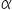yNow if more days are given, fewer binders will be required which means x and z have an inversely proportional relationship i.ex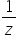So, we can say thatx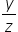x = k…….(1)Where k is a proportionality constantStep 1 of 2:It is given that 18 binders can bind 900 books in 10 days. So,  x = 18, y = 900 and z = 10. Putting the values in equation (1)18 = k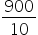k =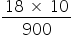=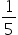Step 2 of 2:Now we are asked to find the number of binders that will be required to bind 660 books in 12 days. Let the number of binders be “b”. So, x = b, y = 660 and z = 12. Putting the values in equation (1)b = k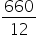Now, put the value of k =d =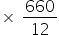d = 11 bindersFinal Answer: Hence, 11 binders will be required to bind 660 books in 12 days.#### With Turito Foundation.#### Get an Expert Advice From Turito.